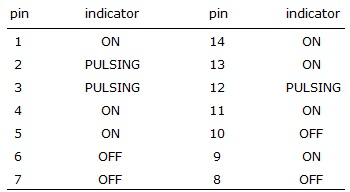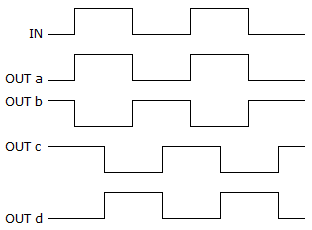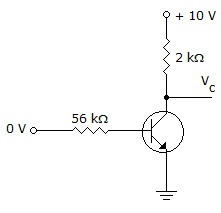# Online Digital Electronics Test - Digital Electronics Test 9Loading Test...

Instruction:

• This is a FREE online test. DO NOT pay money to anyone to attend this test.
• Total number of questions : 20.
• Time alloted : 30 minutes.
• Each question carry 1 mark, no negative marks.
• DO NOT refresh the page.
• All the best :-).

1.

In a binary system there are only two symbols.

A.
 TrueB.
 False2.

The duty cycle of a square wave is ________.

A.
 10%B.
 25%C.
 50%D.
 100%3.

Convert hexadecimal value C1 to binary.

A.
 11000001B.
 1000111C.
 111000100D.
 1110000014.

A logic probe is used to test the pins of a 7411 IC with the following results. Is there a problem with the chip and if so, what is the problem?A.
 Pin 6 should be ON.B.
 Pin 6 should be pulsing.C.
 Pin 8 should be ON.D.
 no problem5.

How many inputs of a four-input AND gate must be HIGH in order for the output of the logic gate to go HIGH?

A.
 any one of the inputsB.
 any two of the inputsC.
 any three of the inputsD.
 all four inputs6.

With regard to a D latch, ________.

A.
 the Q output follows the D input when EN is LOWB.
 the Q output is opposite the D input when EN is LOWC.
 the Q output follows the D input when EN is HIGHD.
 the Q output is HIGH regardless of EN's input state7.

Select the response that best describes the use of the Master Reset on typical 4-bit binary counters.

A.
 When MR1 and MR2 are both HIGH, all Qs will be reset to zero.B.
 When MR1 and MR2 are both HIGH, all Qs will be reset to one.C.
 MR1 and MR2 are provided to synchronously reset all four flip-flops.D.
 To enable the count mode, MR1 and MR2 must be held LOW.8.

A bidirectional 4-bit shift register is storing the nibble 1101. Itsinput is HIGH. The nibble 1011 is waiting to be entered on the serial data-input line. After three clock pulses, the shift register is storing ________.

A.
 1101B.
 0111C.
 0001D.
 11109.

How is a strobe signal used when serially loading a shift register?

A.
 to turn the register on and offB.
 to control the number of clocksC.
 to determine which output Qs are usedD.
 to determine the FFs that will be used10.

What is the difference between a ring shift counter and a Johnson shift counter?

A.
 There is no difference.B.
 A ring is faster.C.
 The feedback is reversed.D.
 The Johnson is faster.11.

A 64-Mbyte SIMM is installed into a system, but when a memory test is executed, the SIMM is detected as a 32-Mbyte device. What is a possible cause?

A.
 The memory module was not installed properly.B.
 The voltage on the memory module is incorrect.C.
 The most significant address line is stuck high or low.D.
 The address decoder on the SIMM is faulty.12.

Which of the following is one of the basic characteristics of DRAMs?

A.
 DRAMs must have a constantly changing input.B.
 DRAMs must be periodically refreshed in order to be able to retain data.C.
 DRAMs have a broader "dynamic" storage range than other types of memories.D.
 DRAMs are simpler devices than other types of memories.13.

What programmable technology is used in FPGA devices?

A.
 SRAMB.
 FLASHC.
 AntifuseD.
 All of the above14.

The PAL structure is able to perform any sum-of-products (SOP) operation.

A.
 TrueB.
 False15.

Of the methods listed, the fastest A/D conversion is done by a ________.

A.
 single-slope ramp converterB.
 dual-slope ramp converterC.
 successive-approximation converterD.
 tracking converter16.

TTL is alive and well, particularly in ________.

A.
 industrial applicationsB.
 educational applicationsC.
 military applicationsD.
 commercial applications17.

Which timing diagram shown below is correct for an inverter?A.
 aB.
 bC.
 cD.
 d18.

Determine the voltage on the collector Vc of the given transistor circuit.A.
 0 VB.
 +10 VC.
 +4.7 VD.
 +0.5 V19.

How many inputs are required for a 1-of-16 decoder?

A.
 2B.
 4C.
 8D.
 1620.

The purpose of a pull-up resistor is to keep a terminal at a ________ level when it would normally be at a ________ level.

A.
 LOW, floatB.
 HIGH, floatC.
 clock, floatD.
 pulsed, float